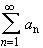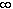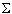#Interactive Real Analysis

Next | Previous | Glossary | Map

## 4.2. Convergence Tests

### Limit Comparison Test

Supposeandare two infinite series. Suppose also that
r = lim | a n / b n |
exists and 0 < r <Thenconverges absolutely if and only ifconverges absolutely.

This test is more useful than the "direct" comparison test because you do not need to compare the terms of two series too carefully. It is sufficient if the two terms behave similar "in the long run".

 Examples 4.2.6:Use the limit convergence test to decide whether the following series converge or diverge. Note that you need to know convergence of the p-series . Does the seriesconverge or diverge ? Does the seriesconverge of diverge ? If r(n) = p(n) / q(n), where p and q are polynomials in n, can you find general criteria for the seriesp(n) to converge or diverge ?
Proof:

Since r = lim | a n / b n | exists, and r is between 0 and infinity there exist constants c and C, 0 < c < C <such that for some positive integer N we have:

c < | a n / b n | < C
if n > N. Assumeconverges absolutely. From above we have that
c | b n | < | an |
for n > N. Hence,converges absolutely by the comparison test.

Assumeconverges absolutely. From above we have that

|a n | < C | b n |
for n > N. But since the series Calso converges absolutely, we can use again the comparison test to see thatmust converge absolutely.Next | Previous | Glossary | Map